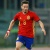IEEE模式分析和机器智能期刊TPAMI（IEEE Trans on Pattern Analysis and Machine Intelligence）出版了有关计算机视觉和图像理解的所有传统领域，模式分析和识别的所有传统领域以及机器智能的选定领域的文章，其中特别强调了用于模式分析的机器学习。还涵盖了诸如可视搜索，文档和手写分析，医学图像分析，视频和图像序列分析，基于内容的图像和视频检索，面部和手势识别以及相关的专用硬件和/或软件体系结构等技术领域。 官网地址：http://dblp.uni-trier.de/db/journals/pami/0+0+0+0+0+0+0+0+0+0+0+0+0+0+0+0+0+0+0+0+0+0+0+0+0+0+0+0+0+0+0+0+0+0+0+0+0+1+0+0+0+0+0+1+0+
Top